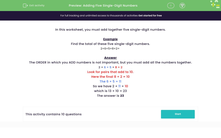### Comprehensive & curriculum aligned

In this worksheet, students add together five single-digit numbers.Key stage:  KS 2

Curriculum topic:   Number: Addition and Subtraction

Curriculum subtopic:   Solve Add/Subtract Problems to 1000

Difficulty level:#### Worksheet Overview

In this worksheet, you must add together five single-digit numbers.

Example

Find the total of these five single-digit numbers.

 2 + 6 + 5 + 8 + 2 =

The ORDER in which you ADD numbers is not important, but you must add all the numbers together.

2 + 6 + 5 + 8 + 2

Look for pairs that add to 10.

Here the final 8 + 2 = 10

The 6 + 5 = 11

So we have 2 + 11 + 10

which is 13 + 10 = 23